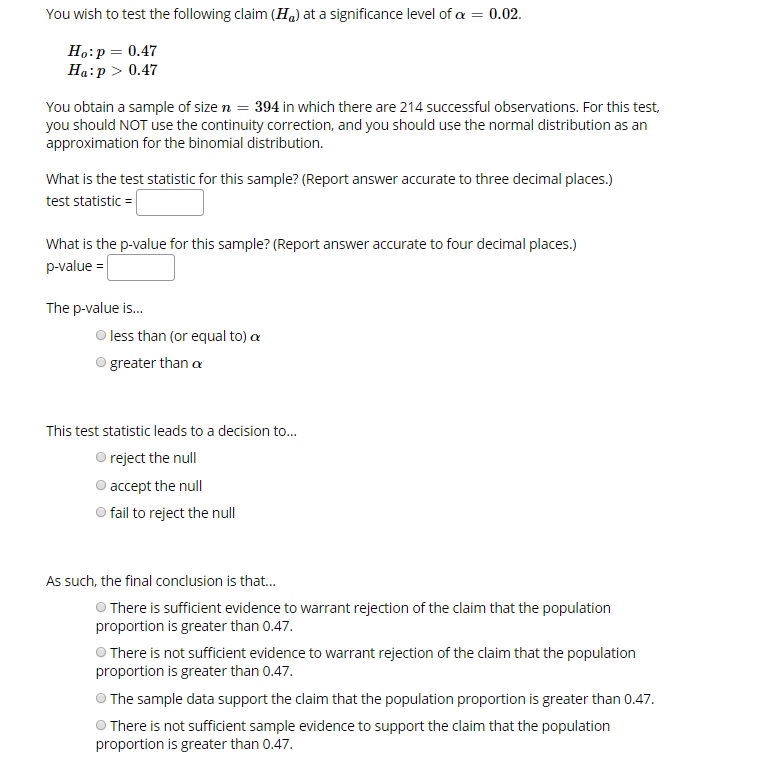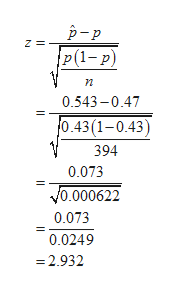# 0.02You wish to test the following claim (H) at a significance level of aHo:p 0.47Ha p 0.47You obtain a sample of size n 394 in which there are 214 successful observations. For this test,you should NOT use the continuity correction, and you should use the normal distribution as anapproximation for the binomial distribution.What is the test statistic for this sample? (Report answer accurate to three decimal places.)test statisticWhat is the p-value for this sample? (Report answer accurate to four decimal places.)p-value =The p-value is...less than (or equal to) agreater than aThis test statistic leads to a decision to...reject the nullaccept the nullfail to reject the nullAs such, the final conclusion is that...There is sufficient evidence to warrant rejection of the claim that the populationproportion is greater than 0.47.There is not sufficient evidence to warrant rejection of the claim that the populationproportion is greater than 0.47The sample data support the claim that the population proportion is greater than 0.47There is not sufficient sample evidence to support the claim that the populationproportion is greater than 0.47

Question
44 viewshelp_outlineImage Transcriptionclose0.02 You wish to test the following claim (H) at a significance level of a Ho:p 0.47 Ha p 0.47 You obtain a sample of size n 394 in which there are 214 successful observations. For this test, you should NOT use the continuity correction, and you should use the normal distribution as an approximation for the binomial distribution. What is the test statistic for this sample? (Report answer accurate to three decimal places.) test statistic What is the p-value for this sample? (Report answer accurate to four decimal places.) p-value = The p-value is... less than (or equal to) a greater than a This test statistic leads to a decision to... reject the null accept the null fail to reject the null As such, the final conclusion is that... There is sufficient evidence to warrant rejection of the claim that the population proportion is greater than 0.47. There is not sufficient evidence to warrant rejection of the claim that the population proportion is greater than 0.47 The sample data support the claim that the population proportion is greater than 0.47 There is not sufficient sample evidence to support the claim that the population proportion is greater than 0.47 fullscreen
check_circle

Step 1

The null and alternative hypotheses are as follows:

From the information, a right tail test with level of significance 0.02 is given.

Null hypothesis:

H0: p = 0.47.

Alternative hypothesis:

Ha: p > 0.47.

From the given information, sample of size (n) 394 there are 214 successful observations.

Step 2

The test statistics is,help_outlineImage Transcriptionclosep-p Z = P(1- p) n 0.543-0.47 0.43(1-0.43) 394 0.073 V0.000622 0.073 0.0249 =2.932 fullscreen
Step 3

The test statistics is (z) is 2.932.

The p-value is:

From standard normal table, the are...

### Want to see the full answer?

See Solution

#### Want to see this answer and more?

Solutions are written by subject experts who are available 24/7. Questions are typically answered within 1 hour.*

See Solution
*Response times may vary by subject and question.
Tagged in

### Other